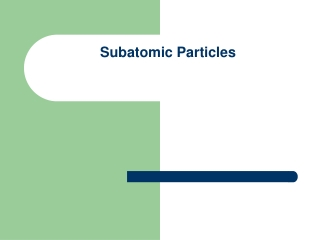DownloadDownload PresentationSubatomic Particles

# Subatomic Particles

Download Presentation## Subatomic Particles

- - - - - - - - - - - - - - - - - - - - - - - - - - - E N D - - - - - - - - - - - - - - - - - - - - - - - - - - -
##### Presentation Transcript

1. Subatomic Particles

2. 1. Subatomic Particles

3. Special notes on subatomic particles • In a neutral atom # of protons=#of electrons. • If the number of protons does not equal the number of electrons you have a charged atom known as an ion. • # protons > # electrons forms a + ion (cation) • # electrons > # protons forms a – ion (anion) • Most of the mass of an atom is in nucleus.

4. Atomic Number (z) • 1913—Henry Mosley • Used to identify an atom • Indicates the number of protons • In a neutral atoms also gives the number of electrons

5. 3. Mass Number (A) • Total number of protons and neutrons in the nucleus of an atom. (nucleons) • Notation: AXZ • Mass # = #protons + # neutrons • # neutrons = Mass # - # protons

6. Try the following:

7. 19F9 9 protons 19-9=10 neutrons 9 electrons 19F9-1 9 protons 10 neutrons 10 electrons 24Mg12 12 protons 24-12=12 neutrons 12 electrons 24Mg12+2 12 protons 12 neutrons 10 electrons Determine the # of protons, neutrons, and electrons for these added examples:

8. Isotopes • Thomson (1912) found 2 types of neon atoms and Soddy (1910) found 2 types of uranium atoms. • 2 elements that have the same atomic number but different mass numbers • Based on atomic structure: 2 elements that have the same number of protons but different number of neutrons. • For example: Cl-35 and Cl-37

9. 5. Atomic Mass • Decimal number • Average of all the naturally occurring isotopes for a particular element • If you round the atomic mass it gives you the mass number for the most common isotope. • Unit is amu (atomic mass unit) • 1 amu is 1/12 the mass of C-12 • Gram atomic mass= amu but in grams

10. Try the following: • Determine the number of protons, neutrons, and electrons for the following isotopes of hydrogen: Solution: Hydrogen-1 (protium) 1H1 1p,0n,1e Hydrogen-2 (deuterium)2H1 1p,1n,1e Hydrogen-3 (tritium) 3H1 1p,2n,1e

11. Naturally occurring chlorine consists of 75% Cl-35 and 25% Cl-37. Find the average atomic mass. • .75(35) + .25(37)= 35.50 amu or • 75(35) + 25(37) =35.50 amu 100

12. 3. Calculate the atomic mass of an element with isotope A occurring 70.0% of the time with a mass of 13.0 amu and isotope B occurring 30.0% of the time with a mass of 15.0 amu. • .70 (13.0) +.30 (15.0) =13.6 amu

13. An element X has three isotopesX-30 has a 50.0% abundance, X-28 has a 30.0% abundance and X-31 has a 20.0% abundance. • .500(30) + .300(28) + .200 (31) = 29.6 amu

14. 5. There are two isotopes of element Z, 60.0% of the atoms have a mass of 58.0 amu and 40.0% have a mass of 57.0 amu. Calculate theatomic mass of element Z. • .600(58.0) + .400(57) = 57.6 amu